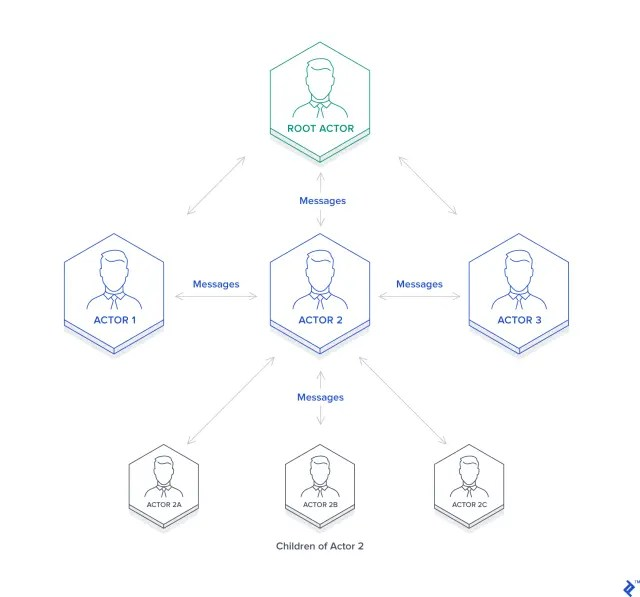# Akka || What is It?

Akka is a toolkit and runtime for building highly concurrent, distributed, and fault-tolerant applications on the JVM. Akka is written in Scala, with language bindings provided for both Scala and Java. The Akka toolkit includes different modules to implement these features. Let’s explore the module Akka Actor to understand how It achieves concurrency.

## How to Set Up Akka Actor in Scala

libraryDependencies += “com.typesafe.akka” % “akka-actor-typed_2.12” % “2.6.6”

## Akka Actor

Akka’s approach to handling concurrency is based on the Actor Model. In an actor-based system, everything is an actor. In the Scala actor system, actors interact and share information, without any presupposition of sequentiality. The way Akka actors share information with one another and task one another is message passing. It uses the Actor model to hide all the thread-related code and gives interfaces to implement a scalable and fault-tolerant system easily.

Let’s see what exactly is an Actor in Akka?

An actor is essentially nothing more than an object that receives messages and takes actions to handle them and It is decoupled from the source of the message so it’s only responsible for properly recognizing the type of message it has received and taking action accordingly.

Upon receiving a message, an actor may take one or more of the following actions:

• Execute some operations itself (such as performing calculations, persisting data, calling an external web service, and so on)
• Forward the message, or a derived message, to another actor
• Instantiate a new actor and forward the message to itTo implement an actor, it is necessary to extend the akka.actor. We extend the Actor trait so as to implement the receive method. Its typical implementation consists of pattern matching. let’s see an example to understand It better :

``````//Sample Code
import akka.actor.Actor
import akka.actor.Props
import akka.event.Logging

class MyActor extends Actor {
case value: String => doSomething(value)
case _ => println("received unknown message")
}
}``````

## How Akka Actors achieve concurrency:

Let’s see a snippet of code so as to understand how Akka Implements concurrency:

``````import akka.actor.{Actor, Props}

case class Add(num1: Int, num2: Int)
case class Subtract(num1: Int, num2: Int)
case class Divide(num1: Int, num2: Int)
class Calculator extends Actor {
case Subtract(num1, num2) => context.actorOf(Props[Subtraction]) ! Subtract(num1, num2)
case Divide(num1, num2) => context.actorOf(Props[Division]) ! Divide(num1, num2)
}
}
case Add(num1, num2) => println(num1 + num2)
}
}
class Subtraction extends Actor {
case Subtract(num1, num2) => println(num1 - num2)
}
}
class Division extends Actor {
case Divide(num1, num2) => println(num1 % num2)
}
}``````

So the output for the code will be:

``````Started Calculating.....